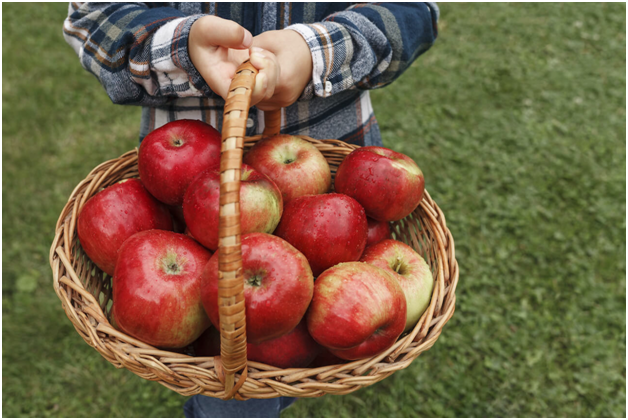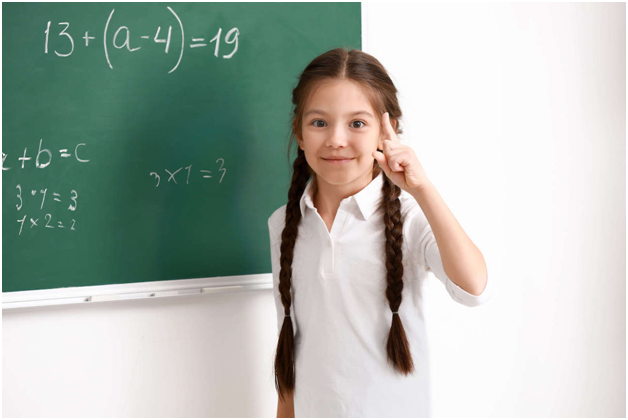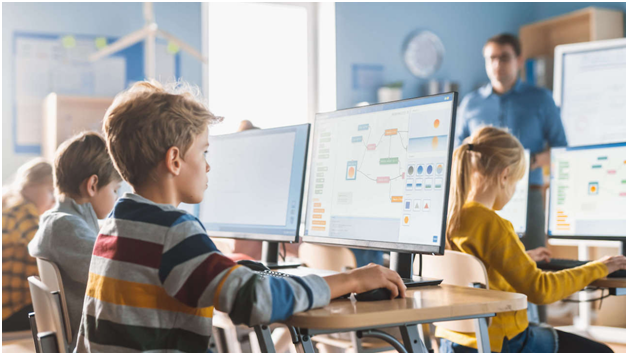# How to Teach Kids Variables

5 viewsAs with many coding basics, variables are all around us in the world, but we rarely refer to them by name. Kids see examples of variables in, for instance, sports — the score is 3 now but it could change to 4 or 6 later. Variables enable them to double the size of a baking recipe or track how many pages they have left in a book.

This implicit ability to use variables is already very powerful. As kids learn more advanced mathematics, they gain a deeper understanding of variables — learning to recognize the ways that they have already been using variables and how to refine their usage for more complicated tasks like what-if scenarios.

This is a necessity for kids to evolve their basic arithmetic skills into important life skills such as setting up a budget.

### Learning Variables for Kids

Understanding how to connect our implicit use of variables with the way that we learn variables in math classes can be difficult. This is where coding can be helpful. Because coding offers an immediate and often visual result when kids play with the values of variables, it offers an easier way for them to understand how variables are working, paving the path for them to understand the mathematics of variables.

• What is a variable?
• How are variables used in math?
• How are variables used in coding?
• How does the usage of variables in coding relate to their usage in math?

## What is a Variable?

The easiest way to explain variables to kids is with real-world examples. The number 3 is a constant. If you say there are 3 apples in a basket, everyone knows exactly how many apples they should expect to see. The number of apples in the basket is 3.But what happens if we add a 4th apple to the basket? The number of apples in the basket is no longer 3. While 3 is a constant, the number of apples in the basket apparently was not! This can happen because the number of apples in the basket is actually a variable. It was 3, but now it has changed to 4. It can even be unknown, but it is always a number.

This is the basic idea that kids learn about variables: you can talk about the number of apples in the basket without actually knowing how many apples that is.

## Variables in MathThe first time kids run across variables is usually in a math class. Variables in math have two parts: a value and a name. Variables are often introduced in math by asking students to solve for the value of a variable, typically x. In earlier pre-algebra math classes, students fill in a blank.

3 + 3 = _

This blank is eventually replaced by x.

3 + 3 = x

The child is asked to solve for x instead of filling in the blank as they used to do. This teaches them to think of variables as a placeholder for an unknown numeric value.

Later, kids are introduced to the concepts of a dependent variable and an independent variable and the idea that variables can have changing values. This allows the introduction of graphs, showing how the value of one variable changes as you adjust other variables.

This eventually evolves into an introduction of science for kids. They can participate in science experiments where, for example, plant growth (the dependent variable) changes as you adjust the amount of sunlight or amount of water flowing to the plant (independent variables) over the course of the experiment.

## How are Variables Different in Coding?

In coding, unlike in math classes, variables should be introduced as objects that can be manipulated rather than just a placeholder for an unknown value. Children should learn that variables can be set or changed by the coder and that some variables affect other variables within the code.

Once they understand what variables are in coding and how to set them, it’s time to introduce how variables can change. Remember, variables in coding aren’t just static values!

Kids can learn to control variables through effect relationships — press the left key and the position of the on-screen character moves to the left. Next, they can learn that the value of a variable can change within the code. For instance, a loop counter will update each time the loop completes.

In coding, kids gain an understanding of variables that shares some similarity to what they learn in math but is more similar to what they learn for scientific experiments. By becoming experimenters with the variables in their code, they gain a deeper understanding of the principles they are learning in their math classes.Thus far, we have talked mostly about how variables overlap in mathematics and coding, but coding also expands the use of variables. Earlier, we mentioned that variables in math have a value and a name. Coding introduces the third part of a variable: type.

While coding, kids will learn to work with different types of variables, each of which has its own purpose. They should understand that strings — which typically contain names or other words — are used differently than integers even though both are stored as variables.

The specific types vary between programming languages, but they will generally learn these basic variable types.

• Integer (…, -2, -1, 0, 1, 2, …)
• Float (1.01, 3.84, 10.0, etc.)
• String (‘worksheet’, ‘high school’, ‘www’, etc.)
• Character (‘a’, ‘b’, ‘c’, …)
• Boolean (true, false)

Variables are a powerful tool for numbers, but by expanding them for use with strings and eventually more complicated types of information, they become a tool with virtually limitless potential! Combine this with the rapid feedback and visual learning that programming provides, and you can see why coding is an ideal companion for mathematics when teaching variables to kids.

## Putting It All Together

Hopefully, this article has given you a better understanding of the similar but distinct ways that variables are used in math and coding. By offering both pathways, you give a child a powerful set of tools to understand and interact with the world around them.

Juni Learning offers courses in both coding and mathematics, empowering your student to get ahead in school, everyday critical thinking, and their future careers. Check out our online coding courses for kids and kids math classes to get started! You can also contact our admissions team for further information.